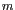DATA MINING
Desktop Survival Guide
by Graham Williams# Imputation

Multiple imputation (MI) is a general purpose method for handling of missing data. The basic idea is: Impute missing values using an appropriate model that incorporates random variation; Do thistimes (often 3-5 times) to obtaindatasets, all with no missing values; Do the intended analysis on each of these datasets; Gert the average values of the parameter estimates across thesamples to have a single point estimate; Calculate standard errors by firstly averaging the squared standard errors of theestimates and calculating the variance of theparameter estimates across samples, and then combine these in some way.

There are a number of R packages for imputation.

Subsections